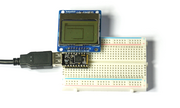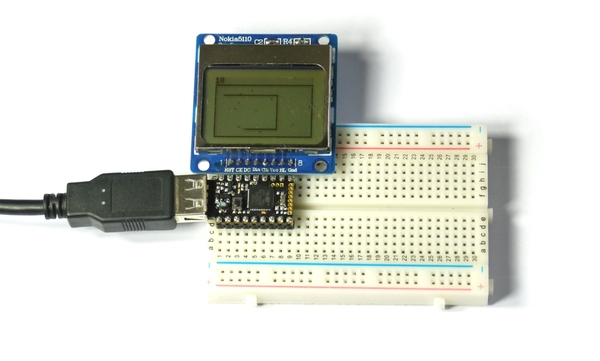# Snake Game

## Introduction

We'll make a very simple snake game using an Espruino Pico and a Nokia LCD display.

## Wiring UpWiring this up is really simple, because the LCD draws so little power that we can power it from Espruino's GPIO lines... Simply place the Espruino Pico on the breadboard with the USB connector facing left, and then place the LCD directly above it, aligned to the right (so the pin on the Pico nearest the USB connector should not be connected to anything). See the picture for an example.

Note: If you wanted to use a normal Espruino board, just follow the instructions on the PCD8544 LCD's page, and update the LCD initialisation code below accordingly.

## Software

We're going to start off using the code from the Nokia 5110 LCD page, but with a few small tweaks:

• We're going to manually set up the pins for the LCD's power
• As the Pico's hardware SPI pins don't align with the LCD, we'll just use Software SPI instead (which will work on any pins).
``````A5.write(0); // GND
A7.write(1); // VCC

// Setup SPI
var spi = new SPI();
spi.setup({ sck:B1, mosi:B10 });
// Initialise the LCD
var g = require("PCD8544").connect(spi,B13,B14,B15, function() {
// When it's initialised, clear it and write some text
g.clear();
g.drawString("Hello",0,0);
// send the graphics to the display
g.flip();
});
``````

Copy and paste this code into the right-hand side of the Web IDE and click the `Send to Espruino` button. The display should now show `Hello`.

Now we're ready to make the snake game. The first step is to make a dot that moves around the screen - copy and paste the following into the right hand side over what was there already:

``````A5.write(0); // GND
A7.write(1); // VCC

// Setup SPI
var spi = new SPI();
// Graphics
var g;
// Snake position and direction
var pos, dir;

function start() {
g.clear();
g.drawRect(0,0,g.getWidth()-1, g.getHeight()-1);
// Setup snake position
pos = {x:g.getWidth()/2,y:g.getHeight()/2}; // centre of the screen
g.setPixel(pos.x, pos.y);
dir = {x:1,y:0}; // the direction of the snake
// update the screen
g.flip();
// start off rendering frames
setInterval(onFrame, 100);
// When a button is pressed, rotate the snake
setWatch(function() { rotate(1); }, BTN, { repeat:true, edge:"rising", debounce: 50});
}

// When Espruino starts up...
function onInit() {
clearInterval();
clearWatch();
spi.setup({ sck:B1, mosi:B10 });
// Initialise the LCD
g = require("PCD8544").connect(spi,B13,B14,B15, function() {
// When it's initialised, clear it and write some text
g.clear();
g.drawString("Hello",0,0);
// send the graphics to the display
g.flip();
start();
});
}

// called every 'frame' of the game
function onFrame() {
pos.x += dir.x;
pos.y += dir.y;
g.setPixel(pos.x, pos.y);
g.flip();
}

function rotate(d) {
if (dir.x) {
dir = {x:0,y:d*dir.x};
} else {
dir = {x:-d*dir.y,y:0};
}
}

// Start everything
onInit();
``````

So now, after it's sent to Espruino you see the 'snake' moving, and you can rotate it in one direction. But what about moving the other way? While we could wire up another Button, let's just make a long press go the other way. Replace `setWatch` at the bottom of the `start` function with the following:

``````setWatch(function(e) {
var duration = e.time - e.lastTime;
if (duration<0.15)
rotate(1);
else
rotate(-1);
}, BTN, { repeat:true, edge:"falling", debounce: 50});
``````

Now, if you just 'dab' the button the snake will turn in one direction, and if you long-press it it'll turn in the other.

So the next step is to detect when the snake bumps into itself. We'll do this by looking at which pixels are set on the screen. Replace `onFrame` with the following, and send it to Espruino again:

``````function gameOver() {
// stop the game
clearWatch();
clearInterval();
// write 'game over' on the screen
g.clear();
var s = "Game Over!";
g.drawString(s, (g.getWidth()-g.stringWidth(s))/2, g.getHeight()/2-4);
g.flip();
// when the button is pressed, restart
setWatch(function(e) {
start();
}, BTN, { edge:"rising", debounce: 50});
}

// called every 'frame' of the game
function onFrame() {
pos.x += dir.x;
pos.y += dir.y;
if (g.getPixel(pos.x, pos.y)) {
gameOver();
} else {
g.setPixel(pos.x, pos.y);
g.flip();
}
}
``````

And now, we have to remember where the snake's tail was previously, so that we can remove the end of it. We'll do it by adding an array called `history` that will contain all the previous positions. This isn't a very efficient way to store the positions, so if this game were to be on a much bigger display with a longer snake then you might need to look at using something else (see the Performance page). However in this case it is good enough and makes the code much easier to understand:

Replace `onFrame` with the following:

``````// previous snake positions
var history = [];
// the length that we'll let the snake get to
var snakeLength = 20;

// called every 'frame' of the game
function onFrame() {
pos.x += dir.x;
pos.y += dir.y;
// remove tail
while (history.length>=snakeLength) {
var p = history.shift(); // remove first item from list
g.setPixel(p.x, p.y, 0); // clear that pixel
}
// add current position onto the end
history.push({x:pos.x, y:pos.y});

if (g.getPixel(pos.x, pos.y)) {
gameOver();
} else {
g.setPixel(pos.x, pos.y);
g.flip();
}
}
``````

And finally, we'll want to add some 'apples' that the snake can eat, some scoring, and we're good to go.

Apples are stored in an array called `apples`, and now, when the snake bumps into something we check to see if it's an apple - and if it was we add to the score rather than ending the game.

The finished game code is as follows:

``````A5.write(0); // GND
A7.write(1); // VCC

// Setup SPI
var spi = new SPI();
// Graphics
var g;
// Current score
var score = 0;
// Snake position and direction
var pos, dir;
// previous snake positions
var history = [];
// the length that we'll let the snake get to
var snakeLength = 20;
// a list of apple locations (that the snake can eat)
var apples = [];

function drawScore() {
// remove old score...
g.setColor(0);
g.fillRect(0,0,g.getWidth(),5);
g.setColor(1);
g.drawString(score);
}

function newApple() {
var p;
// keep coming up with random locations until there
// is nothing drawn where we want to put the apple
do {
p = { x : Math.round(Math.random()*g.getWidth()),
y : 6 + Math.round(Math.random()*(g.getHeight()-6)) };
} while (g.getPixel(p.x, p.y));
// draw the apple, and save it inthe array
g.setPixel(p.x, p.y);
apples.push(p);
}

function start() {
// clear screen
g.clear();
g.drawRect(0,6,g.getWidth()-1, g.getHeight()-1);
// reset score
score = 0;
drawScore();
// Setup snake position
pos = {x:g.getWidth()/2,y:g.getHeight()/2}; // centre of the screen
history = pos.x, pos.y; // reset the 'history' list
g.setPixel(pos.x, pos.y);
dir = {x:1,y:0}; // the direction of the snake
// Now add randomly positioned apples
apples = [];
for (var i=0;i<10;i++)
newApple();
// update the screen
g.flip();
// start off rendering frames
setInterval(onFrame, 100);
// When a button is pressed, rotate the snake
setWatch(function(e) {
var duration = e.time - e.lastTime;
if (duration<0.15)
rotate(1);
else
rotate(-1);
}, BTN, { repeat:true, edge:"falling", debounce: 50});
}

// When Espruino starts up...
function onInit() {
clearInterval();
clearWatch();
spi.setup({ sck:B1, mosi:B10 });
// Initialise the LCD
g = require("PCD8544").connect(spi,B13,B14,B15, function() {
// When it's initialised, clear it and write some text
g.clear();
g.drawString("Hello",0,0);
// send the graphics to the display
g.flip();
start();
});
}

function gameOver() {
// stop the game
clearWatch();
clearInterval();
// write 'game over' on the screen
g.clear();
var s = "Game Over!";
g.drawString(s, (g.getWidth()-g.stringWidth(s))/2, g.getHeight()/2-4);
g.flip();
// when the button is pressed, restart
setWatch(function(e) {
start();
}, BTN, { edge:"rising", debounce: 50});
}

// called every 'frame' of the game
function onFrame() {
pos.x += dir.x;
pos.y += dir.y;
// remove tail
while (history.length>=snakeLength) {
var p = history.shift(); // remove first item from list
g.setPixel(p, p, 0); // clear that pixel
}
// add current position onto the end
history.push([pos.x, pos.y]);

if (g.getPixel(pos.x, pos.y)) {
// check for apples
var wasApple = false;
for (var i in apples)
if (apples[i].x==pos.x && apples[i].y==pos.y) {
wasApple = true;
// delete this apple
apples.splice(i,1);
// add a new apple
newApple();
// change score and increase snake length
snakeLength += 5;
score += 10;
drawScore();
// break out so we don't check any more apples
break;
}
if (!wasApple)
gameOver();
} else {
g.setPixel(pos.x, pos.y);
g.flip();
}
}

function rotate(d) {
if (dir.x) {
dir = {x:0,y:d*dir.x};
} else {
dir = {x:-d*dir.y,y:0};
}
}

onInit();
``````

This page is auto-generated from GitHub. If you see any mistakes or have suggestions, please let us know.